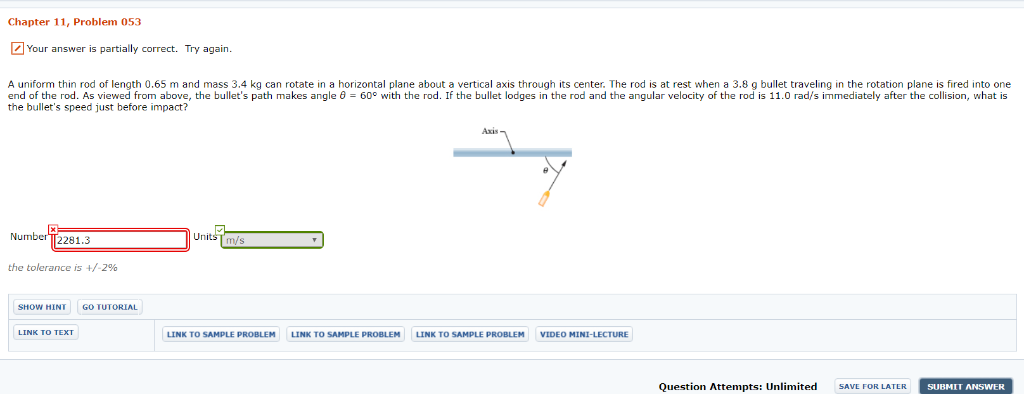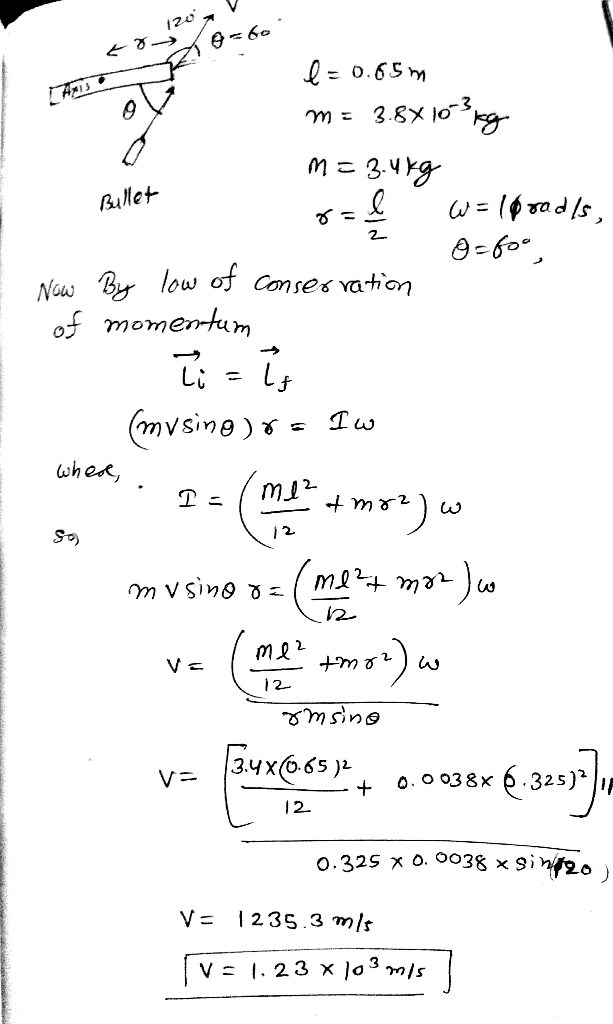# A uniform thin rod of length 0.65 m and mass 3.4 kg can rotate in a horizontal plane about a vert...

A uniform thin rod of length 0.65 m and mass 3.4 kg can rotate in a horizontal plane about a vertical axis through its center. The rod is at rest when a 3.8 g bullet traveling in the rotation plane is fired into one end of the rod. As viewed from above, the bullet's path makes angle θ = 60° with the rod. If the bullet lodges in the rod and the angular velocity of the rod is 11.0 rad/s immediately after the collision, what is the bullet's speed just before impact?Chapter 11, Problem 053 Your answer is partially correct. Try again. A uniform thin rod of length 0.65 m and mass 3.4 kg can rotate in a horizontal plane about a vertical axis through its center. The rod is at rest when a 3.8 g bullet traveling in the rotation plane is fired into one end of the rod. As viewed from above, the bullet's path makes angle θ 60° with the rod. If the bullet lodges in the rod and the angular velocity of the rod is 11.0 rad/s immediately after the collision, what is the bullet's speed just before impact? Number T2281.3 the tolerance is +/-296 SHOW HINT GO TUTORIAL LINK TO SAMPLE PROBLEM LINK TO SAMPLE PROBLEMLINK TO SAMPLE PROBLEM VIDEO MINI-LECTURE Question Attempts: Unlimited SAVE FOR LATER SUBMIT ANSWER##### Add Answer of: A uniform thin rod of length 0.65 m and mass 3.4 kg can rotate in a horizontal plane about a vert...
More Homework Help Questions Additional questions in this topic.